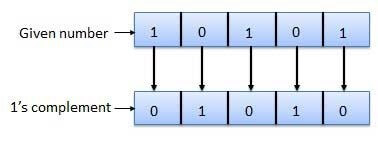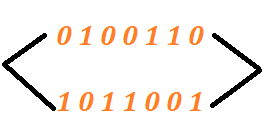# One’s complement calculator

Enter binary value in the input box below and click the Calculate button to find the 1s complement using one’s complement calculator.1s complement calculator calculates the 1’s complement of the given binary number in fraction of seconds. Use this tool to convert a binary number into one’s complement.

## What is one’s complement?

Ones' complement of a binary number is the value obtained by inverting all the bits in the binary representation of the number by swapping 0s and 1s.

It is mostly used in computer science, where it has varying effects depending on how a specific computer represents numbers. In most cases, 1’s complement is followed by two’s complement.## How to find one’s complement?

To find ones complement without using 1’s complement converter, follow the example below.

Example:

Convert (0100110)2 to one’s complement.

Step 1: Write down the binary number. If you have a decimal number, then convert decimal to binary number first.

(0100110)2

Step 2: Swap each 0 with 1 and each 1 with 0. (Invert all values).

After swapping, we got:(0100110)2 = 1011001

1. One’s Complement. by tutorialspoint.com